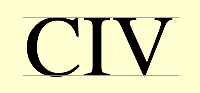Roman Numerals 4
Set 4 (10 Questions)
Students learn the value of the various Roman Numerals. Students compare the value of Roman and standard numerals. Students have access to links about the historical use of Roman Numerals. Again, there are slightly larger values than sets 1, 2 & 3.
From Mr. Anker Tests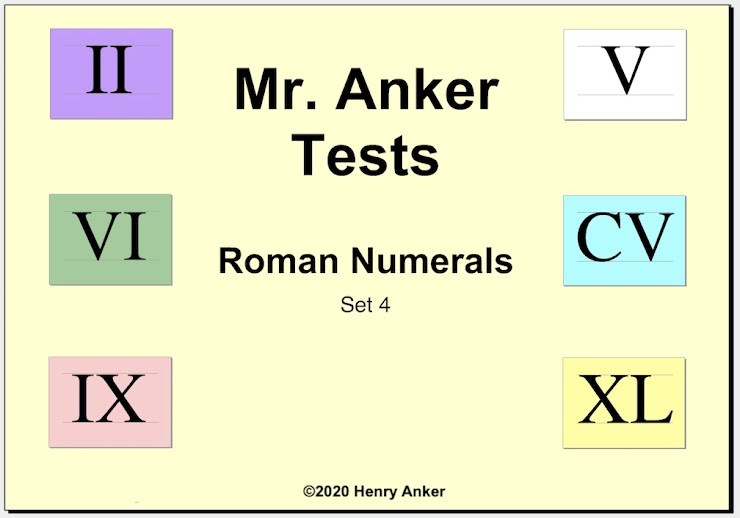Roman Numerals Help Slide 1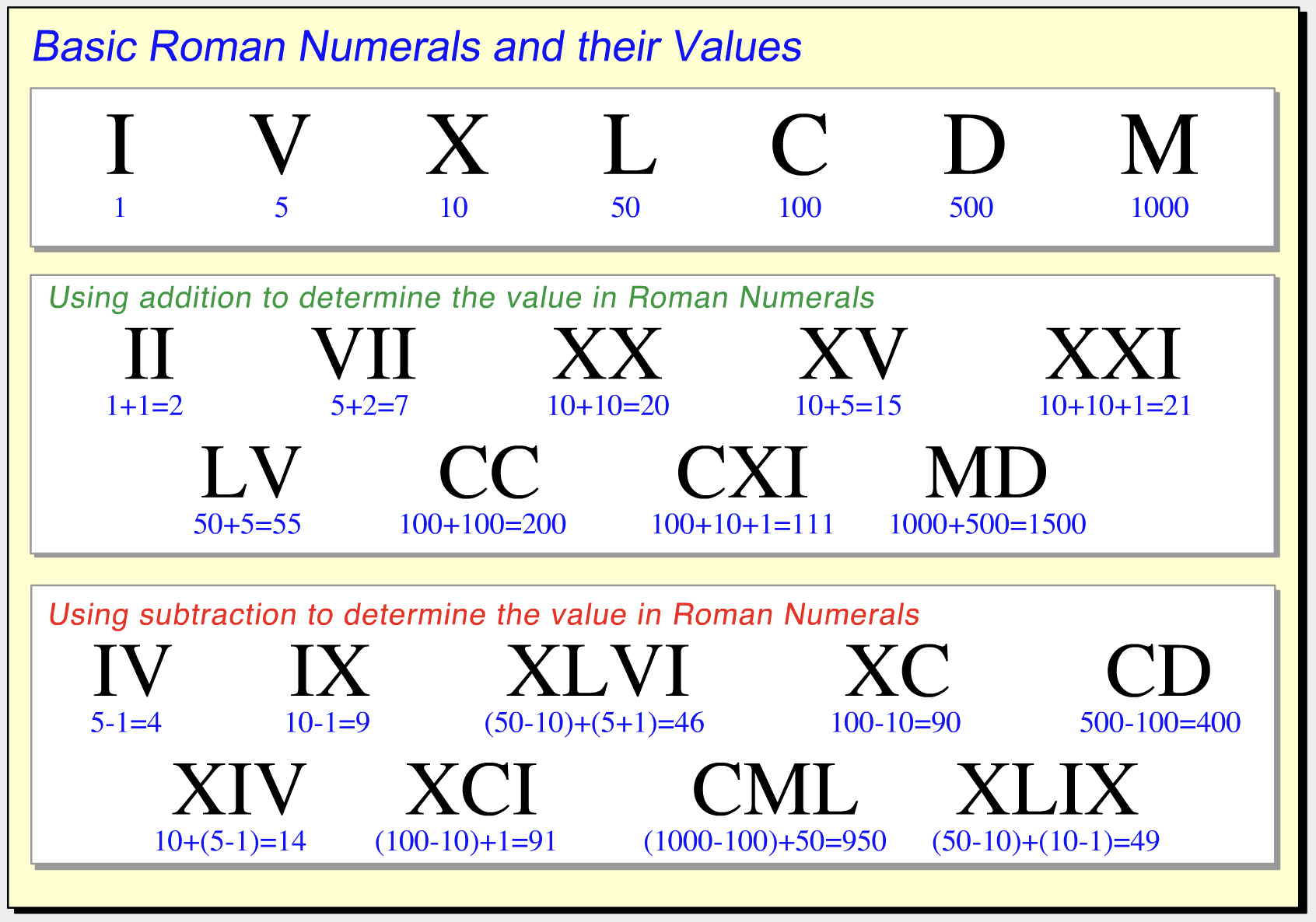This outstanding video can be found on Socratica Math
Roman Numerals Help Slide 2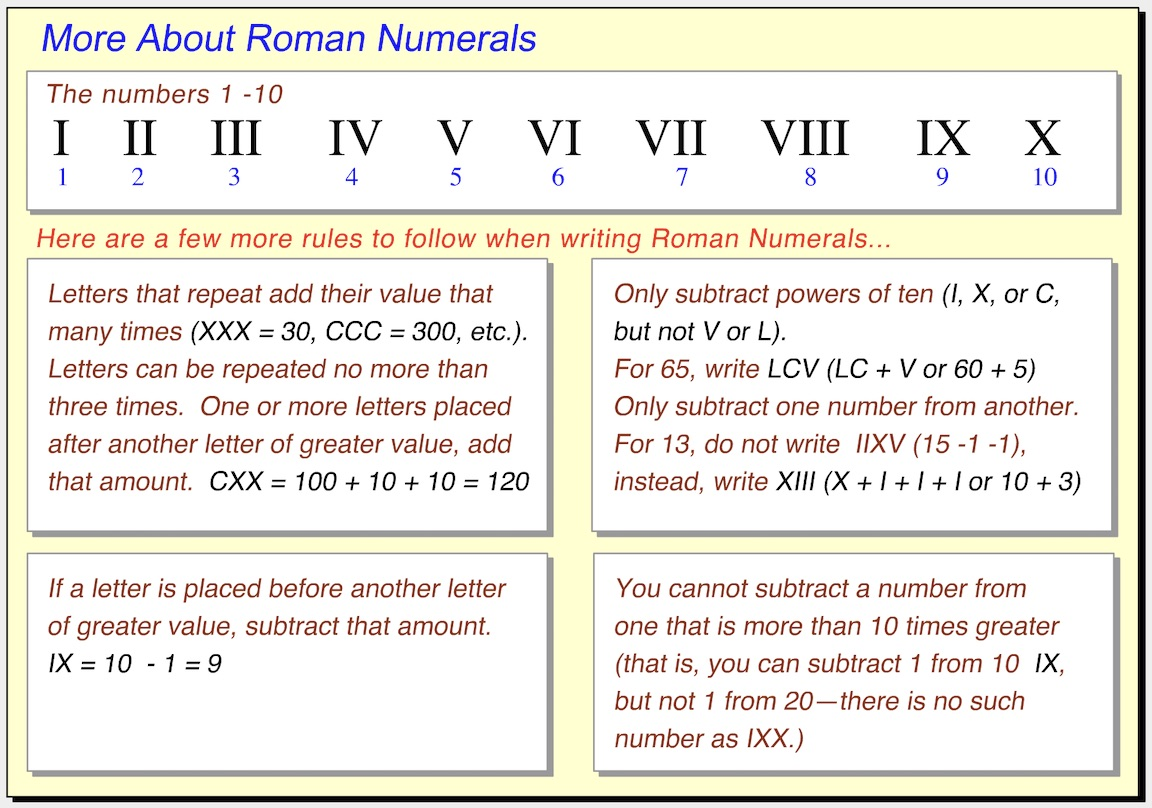1. The standard numeral 30 is ____________ the Roman Numeral XXIX. *
1 point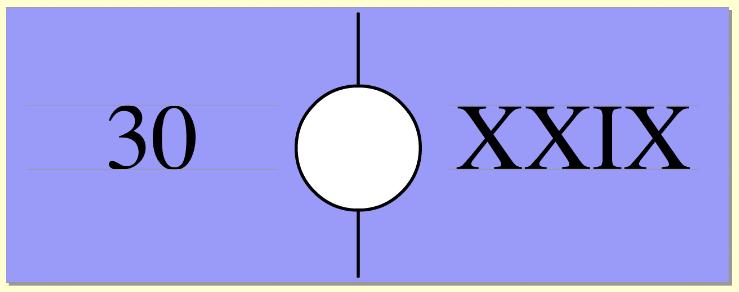2. Match the standard numeral to this Roman Numeral. *
1 point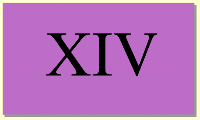3. The standard numeral 35 is ____________ the Roman Numeral LV. *
1 point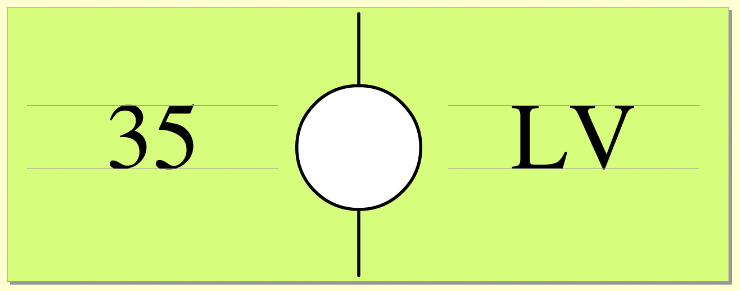4. Match the standard numeral to this Roman Numeral. *
1 point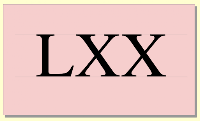5. The standard numeral 47 is ____________ the Roman Numeral XLVII. *
1 point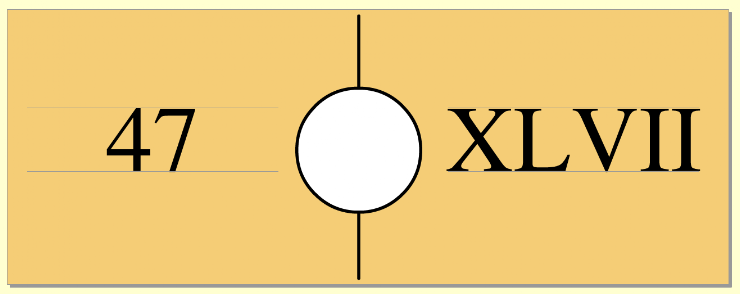6. Match the Roman Numeral to this standard numeral. *
1 point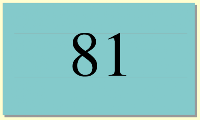7. The Roman Numeral C is ____________ the standard numeral 100. *
1 point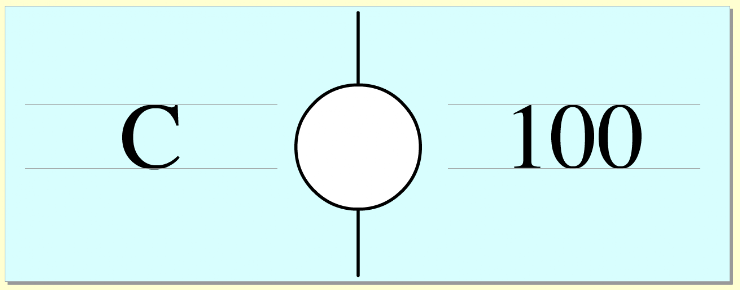8. Match the Roman Numeral to this standard numeral. *
1 point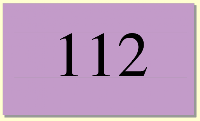9. Enter, by typing, the standard numeral for this Roman Numeral. *
1 point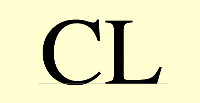10. Enter, by typing, the standard numeral for this Roman Numeral. *
1 point